# Iterators, containers and algorithms

The C++ Standard Library includes the Standard Template Library (STL), that is a collection of generic algorithms, data structures (containers) and iterators.
Although STL and Standard Library are formally different things, C++ programmers generally consider STL and Standard Library as the same thing. Practically speaking, the Standard Library has been developed following the design principles of the STL.

In the following article we'll use the terms "STL" and "Standard Library" referring to the same thing.

Today we'll meet the triad of the STL: iterators, containers and algorithms.

## Containers

A container is a generic class which implements a certain data structure.
Reference: http://en.cppreference.com/w/cpp/container

A container:

• is generic, meaning that it works on whatever data type satisfying certain basic requirements (formally: Concepts) needed by the container (not satisfying is formally undefined behavior - generally the compiler complains);
• manages how the elements are stored;
• satisfies some requirements (Concepts);
• provides some functions to operate on the stored elements and on itself.

The basic Concept that every container has to implement is, just, Container. On top of this, containers can implement others. For instance:

vector<T>


Is also a ContiguousContainer (until C++14 it was just a SequenceContainer), ReversibleContainer e AllocatorAwareContainer.

In the STL, containers are categorized as:

• Sequence containers (some are also contiguous)
• Associative containers
• Unordered associative containers

Every container stores and manages objects of a certain type. For example:

vector<int>


Contains int values. This type is called underlying type and can be accessed through:

vector<int>::value_type


Every container provides a value_type because the concept Container requires such type definition.

Examples:

string s; // typedef per basic_string<char>
vector<int> v;


For T to be used as an underlying type is required to implement some concepts that can vary from container to container. Some depend also on the operations the user is going to perform with the container. For example, you can use a vector with moveable type that is not copyable as far as you don't try to copy the container.

The basic concept is Erasable, that is the possibility to be erased through a certain allocator. By default (by using std::allocator) this means that the underlying type has a public destructor.

### An example: sum of a sequence

Let's meet std::vector: we have to print the sum of the elements of a sequence.

std::vector is likely the simplest data structure to use for this task, it's just kind of a dynamic array:

void PrintSum(const vector<int>& v)
{
int sum = 0;
for (auto i=0u; i<v.size(); ++i)
sum += v[i]; // i-th position (0-based)
cout << sum;
}


We used:

• size(), to have back the numbero of elements really contained,
• operator[i], to have back a reference to ith stored element.

Some important facts about std::vector:

• guarantees a contiguous storage (we can even access such storage by pointer - e.g. to pass it to a C API);
• manages automatically the length (size) and the capacity (capacity) of the storage;
• it's possible to both preallocate (reserve) and preinitialize (resize) a vector;
• adding an element to a vector may result in relocating its elements (to maintain the contiguity);

Let's say we want to replace std::vector with std::list, that is a doubly linked list, providing insertions and removals in constant time:

void PrintSum(const list<int>& l)
{
int sum = 0;
for (auto i=0u; i<l.size(); ++i)
sum += l[i]; // ?
cout << sum;
}


GCC complains:

prog.cc:46:20: error: no match for 'operator[]' (operand types are 'const std::__cxx11::list<int>' and 'int')
sum += l[i]; // ?


Basically, list is not providing operator[] as vector.

To understand how to access list, we need to introduce the second element of the triad: the iterator.

## Iterators

An iterator is an object which hides how (the internal details) to iterate and access the elements on a certain data structure.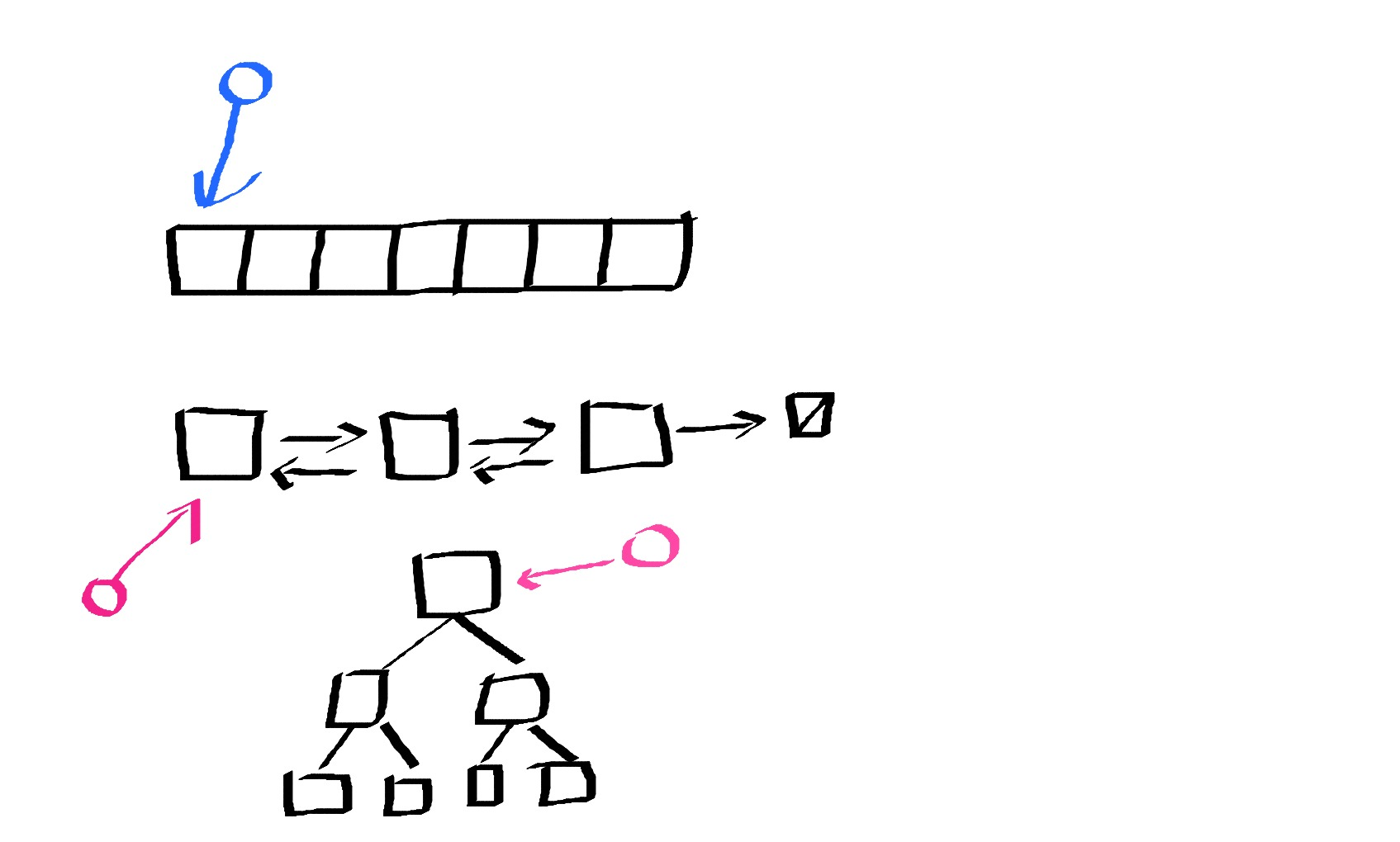Is it possible to print every element of the data structure above by using the same syntax?

Ideally, we'd like writing:

void print(iterator it)
{
while (it.hasNext())
cout << it.Next();
}


First of all, in C++ we always pass two iterators to identify a range of elements:

void print(iterator begin, iterator end)
{
while (begin != end)
...
}


[begin, end) is a half open range, that is elements are from begin to end excluded. For example, for a vector: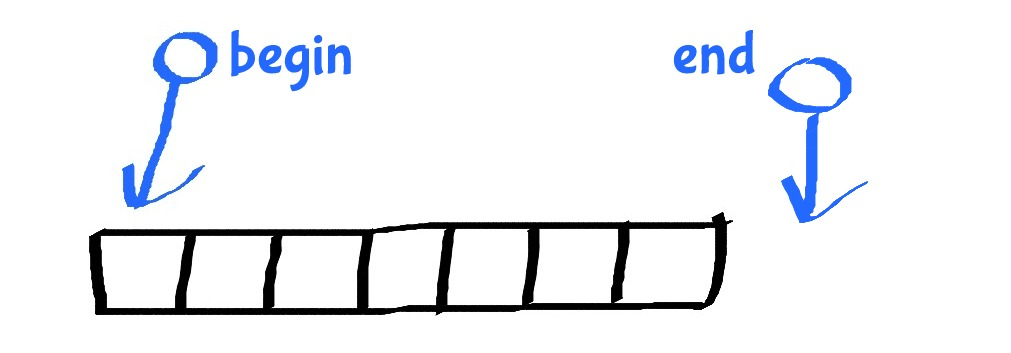Advantages are mainly two:

• no special case for the empty range (begin is equal to end in this case);
• loop termination condition is simply begin != end.

Considering the previous picture, to print only the first 4 elements of the vector, here is the begin, end pair to pass to print: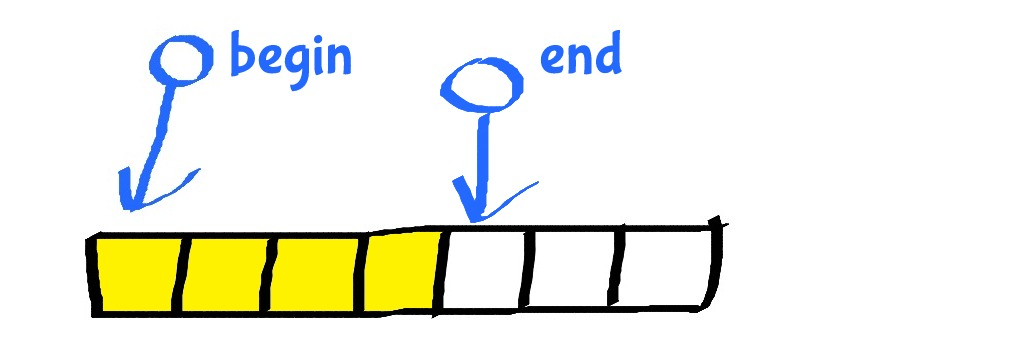STL containers make it easy to obtain the begin and the end iterators through begin() and end() member functions:

vector<int> v = {...};
print(v.begin(), v.end());


To put our print in production, we have to understand how we can access the underlying element of an iterator and how to move the iterator forward

Iterator syntax is similar to pointers syntax

To obtain a reference to the underlying element, we dereference the iterator:

auto& value = *it;


To move the iterator forward by one position, we increment the iterator:

++it; // oppure it++


The difference between pre-increment (++it) and post-increment (it++) is subtle: in the latter case, the operator++ returns a copy to the iterator before the move.

Let's complete the function print:

void print(iterator begin, iterator end)
{
while (begin != end)
{
cout << *begin;
++begin;
}
}


In C++, an iterator can be any type behaving as an iterator. We can pass to print, any object supporting:

• an operator to compare - !=;
• an operator to dereference - *;
• an operator to increment - ++.

Actually, we can even use a couple of plain pointers:

int arr[] = {1,2,3};
print(arr, arr + 3);


Idiomatically in C++ we write:

print(begin(arr), end(arr));


Since C++11, the STL has provided std::begin and std::end (and const/reverse variants) that for a standard container is exactly container.begin() and container.end(), but they also work with C arrays. It's always preferred to use the non-member version of begin/end rather than the corresponding member functions, just because they are more generic.

Let's print the first four elements, again:Remembering C pointers arithmetic, we try:

vector<int> v = {...};
print(begin(v), begin(v)+4);


And it works!

We try with list:

list<int> l = {...};
print(begin(l), begin(l)+4);


However we get:

error: invalid operands to binary expression ('decltype(__c.begin())' (aka '__list_iterator<int, void *>') and 'int')


It means that list iterator cannot be added to an int.

It's time to introduce another important idea behind standard iterators: the iterator category.

In C++ iterators have different categories depending on the “class of operations” they are able to do. Such categories are stated by the standard: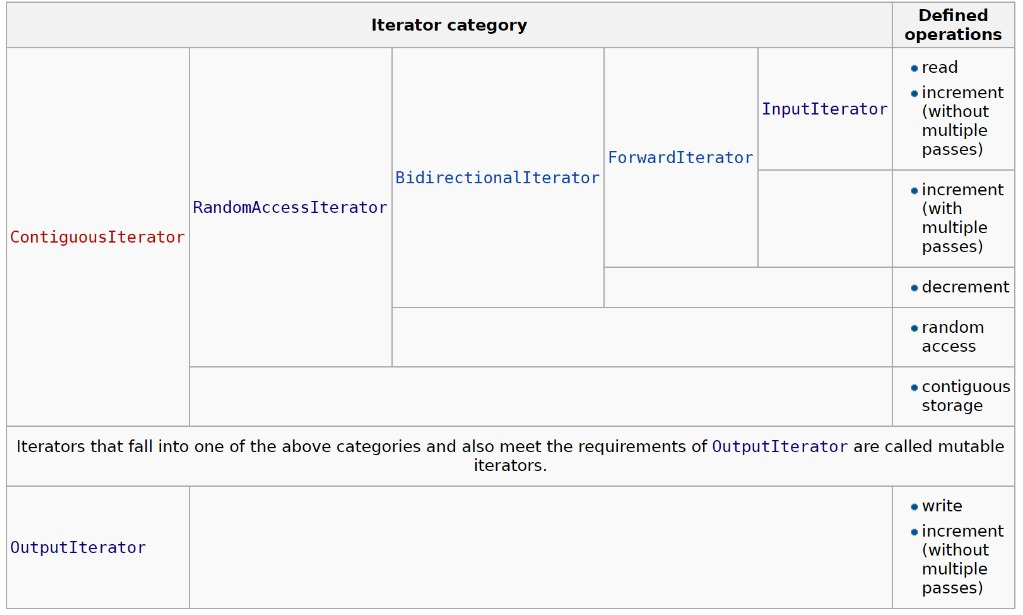The organization of the categories in the image above is not accidental: apart from OutputIterator, the others are displayed from the most “powerful” (ContiguousIterator) to the least (InputIterator). An iterator of a certain category provides the whole set of operations of the lower categories. For instance, a BidirectionalIterator can be:

• read (e.g. *it);
• incremented (e.g. ++it);
• decremented (e.g. --it).

However, it does not provide random access (e.g. it + N or it[N]) nor it can guarantee that it will store elements contiguously.

This way, any type supporting operations of a certain category can be used as an iterator.

In our previous example, list iterator is not a BidirectionalIterator and so it cannot be randomically accessed (e.g. it + 4 is not supported). Note: it does not make sense to support such operation because moving a list iterator by N positions requires N steps, that is a linear time. Instead, random access should be constant.

To have the same result we can do:

list<int> l = {...};
auto fourthEnd = begin(l); ++fourthEnd; ++fourthEnd; ++fourthEnd; ++fourthEnd;
print(begin(l), fourthEnd);


However, it's not very handy.

Let's resort to the standard and use std::next, that will return a given iterator incremented by N steps:

list<int> l = {...};
print(begin(l), next(begin(l), 4));


If an iterator is RandomAccess, such operation just does +N on the iterator, whereas it will end up with incrementing the iterator N times in the other cases.

We are stating that any type able to provide a certain iterator category operations can be used as an iterator of that category. Functions will “expect” on categories rather than on types. For our print an InputIterator is enough because we just need to access the range in a single pass.

Other functions may have higher requirements. For instance, a binary search (e.g. std::binary_search, std::lower_bound) requires a ForwardIterator because the algorithm operates in a multi-pass fashion (e.g. reading an element at position i may also require to step back and read an element at position i-j):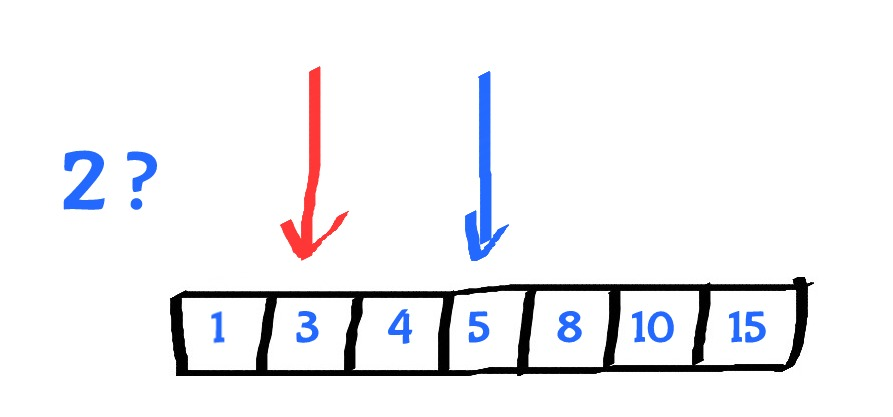Another interesting example consists in reading the standard input. The principle is very simple and is consistent with the philosophy of the STL: we can still use iterators that will hide the standard input instead of a container:

print(istream_iterator<int>(cin), istream_iterator<int>());


An istream_iterator reads successive objects of type T from the std::basic_istream object for which it was constructed, by calling the appropriate operator>>. The default-constructed std::istream_iterator is the end-of-stream iterator.

Now, let's say we want to write to another stream, not cout by default. We can redesign our function this way:

void print(Iterator begin, Iterator end, Iterator out)
{
while (begin != end)
{
*out = *begin;
++begin;
++out;
}
}


Reading carefully, we realize that the expression *it = o is not required on InputIterator category. Instead, we should expect that out is an OutputIterator:

void print(InputIterator begin, InputIterator end, OutputIterator out)
{
while (begin != end)
{
*out = *begin;
++begin;
++out;
}
}


Let's use an ostream_iterator, to emulate what we were doing before this refactoring:

print(  istream_iterator<int>(cin), istream_iterator<int>(),
ostream_iterator<int>(cout));


The name print is wrong. This function is able to do something more generic: it copies a range begin, end starting from out. The standard library already provides std::copy.

Give a warm welcome to our first algorithm.

## Algorithms

An algorithm is a generic function doing a certain operation on (at least) a range of elements [begin, end).

Now we can unveal the type of an iterator: it's basically a template:

template<typename InputIt, typename OutputIt>
OutputIt copy(InputIt first, InputIt last, OutputIt d_first);


By convention, the STL gives names to template parameters as the names of the required categories.

An algorithm never changes the storage of a containers, clearly because it ignores what's underlying a iterators range. Afterward we'll understand the idioms to combine algorithms and mutable operations like removing elements.

The STL provides several algorithms:
http://en.cppreference.com/w/cpp/algorithm

Why it's preferable to use an algorithm instead of a hand-made for loop:

• efficiency: algorithms can easily employ low-level optimizations (e.g. std::copy on PODs is actually a memcpy);
• correctness: custom code comes with more opportunities to make mistakes;
• maintainability: algorithms are standard. This means: well-known and scoped;
• composability: you can combine more algorithms in one call. This will be even more clear with ranges in C++20. Start using standard algorithms today will make it easier to exploit the whole power and flexibility of ranges tomorrow.

To get familiar and fluent with algorithms you should practice with them.

Apart from our daily job, competitive programming gives such opportunity (e.g. HackerRank).

## Challenges

### Simple array sum

Problem: given a sequence in input, print its sum.
Challenge on HackerRank

Step by step:

Let's start from a previous example we coded:

void PrintSum(const vector<int>& v)
{
int sum = 0;
for (auto i=0u; i<v.size(); ++i)
sum += v[i];
cout << sum;
}


First question to answer: does it exist a standard algorithm doing what we are crafting by hand?

Yes: std::accumulate.

std::accumulate aggregates a sequence by using a binary operation (by default is the simple sum):

auto aggregatedValue = accumulate(begin, end, startValue);


We can replace our loop with accumulate:

void PrintSum(const vector<int>& v)
{
cout << accumulate(begin(v), end(v), 0);
}


Now we should read the vector from the standard input. The input format is trivial: first we get the number of elements, then the elements space-separated:

5
1 5 4 2 6


We can use std::copy, as we did before:

int n; cin >> N; // numero di elementi
vector<int> n(N); // resize (mette tutti gli elementi a 0)
copy(istream_iterator<int>(cin), istream_iterator<int>(), begin(v))


However, what if our exercise required to read another vector? E.g.:

5
1 5 4 2 6
4
1 2 3 4


Our code won't work. We should bound to N our read (copy) operation.

The standard provides a second version of copy that does what we need, copy_n:

copy_n(istream_iterator<int>(cin), N, begin(v));


copy and copy_n skips the separators (by default: spaces and carriage returns).

Another improvement consists in not reading the input at all. We can actually just use accumulate on istream iterators:/p>

accumulate(istream_iterator<int>(cin), istream_iterator<int>(), 0);


However, we should skip the first number, that is the size of the vector that will be passed. A simple way to do that consists in using std::next:

cout << accumulate(next(istream_iterator<int>(cin)), istream_iterator<int>(), 0);


### camelCase

Problem: count the number of words in a string written in camelCase.
Challenge on HackerRank

Step by step:

We should count the number of uppercase letters and then sum 1 (the first is lowercase).

counting means that we can try employing these ones: count and count_if.

count returns the number of occurrences of an element in a certain range, count_if returns the number of elements for which a certain predicate is true.

We can use count_if returning true if a letter is uppercase. Let's use a lambda expression to define our predicate inline:

[](char c) { return isupper(c); }


Solution:

cout << (count_if(istream_iterator<char>(cin), istream_iterator<char>(), [](char c){
return isupper(c);
})+1);


### Missing element

Problem: given two sequences, the second is the same as the first except for one element. Find such element.

There are many solutions.

We use a xor operations to avoid both additional storage and possible interger overflow:

const auto bs_xor = accumulate(begin(baseline), end(baseline), 0, bit_xor<>());
const auto ac_xor = accumulate(begin(actual), end(actual), 0, bit_xor<>());
cout << (bs_xor ^ ac_xor) << endl; // the missing number


std::accumulate is flexible enough to allow custom aggregate operations. We used std::bit_xor, a standard function object. Standard function objects are, just like algorithms, a - simple - collection of well-known and scoped “callable” objects.

### Is Palindrome

Problem: given a string, determine if it's palindrome.

We can verify if the string is the same as itself read in the opposite direction:

string s; cin >> s;
bool isPalindrome = true;
for (auto i=0u; isPalindrome && i<s.size(); ++i)
{
isPalindrome = (s[i] == s[s.size()-i-1])
}
cout << (isPalindrome ? "YES" : "NO");


The STL provides std::equal which verifies if two ranges are the same. We can use it as far as we understand how to pass iterate the string the other way.

Normal iterators can have counterparts called reverse iterators. They enable us to iterate ranges in the opposite direction:

std::equal(begin(s), end(s), rbegin(s));


This solution is still not perfect. Let's analyse our comparisons one by one:

s = "abcdcba"
N = s.size(); // 7

s == s[N-1] ==> s
s == s[N-2] ==> s
s == s[N-3] ==> s
s == s[N-4] ==> s
s == s[N-5] ==> s
s == s[N-6] ==> s
s == s[N-7] ==> s


s == s is the last mandatory control, the others have already been performed. We should do something like: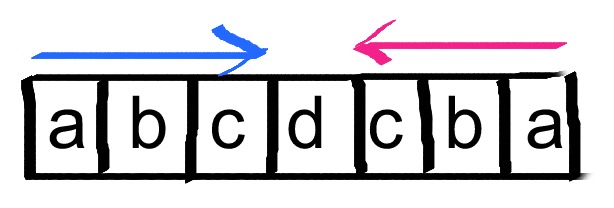We should verify only the first begin(s)+N/2 elements:

std::equal(begin(s), begin(s)+s.size()/2, rbegin(s));


Or, similarly:

std::equal(begin(s), next(begin(s), s.size()/2), rbegin(s));


### Starts with

Problem: determine if a std::string starts with another given string (a prefix).
Example: "www.conoscerelinux.org" starts with "www."? Yes.

This exercise gives us an opportunity to meet std::string functions like:

auto startsWithPrefix = s.compare(0, prefix.size(), prefix) == 0;


### Alternating Characters

Problem: given a string, find the minimum amount of characters to remove such that the string does not contain equal adjacent characters anymore.
Example: "ABAA", 1 (just remove one 'A' at the end).
Challenge on HackerRank

We can just use how many adjacent characters are equal:

string s;
cin >> s;
auto cnt = 0;
for (auto i=1; i<s.size(); ++i)
{
if (s[i]==s[i-1])
cnt++;
}
cout << cnt;


Let's explore the standard library and find other solutions.

The for loop is doing something like: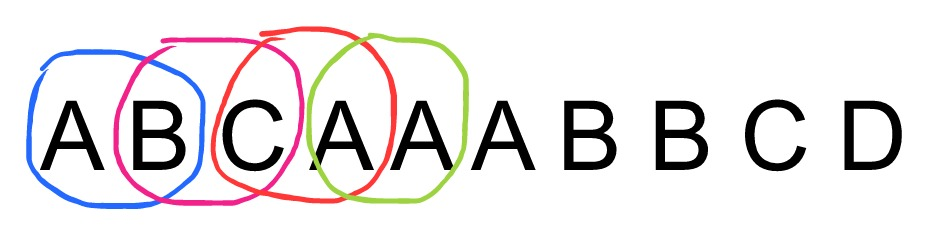Now, we can visualize the algorithm from another point of view:The second string is actually the first one shifted by one position. From this point of view we are turning the problem into: counting the number of equal pairs. We could use count_if if the standard had something like adjacent_element_iterator.

Thinking more about the algorithm, we have two operations involved:

• comparing each pair
• summing the number of times the comparison is true

Forget the problem for a moment and replace characters with numbers:

  1 2 3 4 5
1 2 3 4 5


Instead of comparing, let's do a product:

res = 1*2 + 2*3 + 3*4 + 4*5


This is a scalar product (or dot product or, innter product). The STL has std::inner_product performing this operation. The STL is flexible and this algorithm allow us to customize “sum” e “product”. Instead of the mathemtical product we just need the comparison, which returns 1 or 0:

cin >> s;
cout << inner_product(begin(s), end(s)-1, next(begin(s)), 0, plus<>(), equal_to<>());

In C++, inner_product can be used whatever a zip_with is needed. This idiom is unknown by many people.

The other solution comes from a simple idea: suppose we want to remove the adjacent equal characters.

What's the point with remove_if and remove? Before we told that algorithms cannot mutate the internal storage of a container. E.g. in this case, how remove can physically remove elements from the string?

They do not remove elements, they just overwrite elements to remove with the one sto maintain, such that at the end of the structure some contiguous holes will be left. Such holes will be physically erased by the container.

For instance, suppose we remove elements bigger than 14 from this vector:remove_if just overwrites (technically move-assigns, if possible, or just assigns if not) elements to remove with good ones: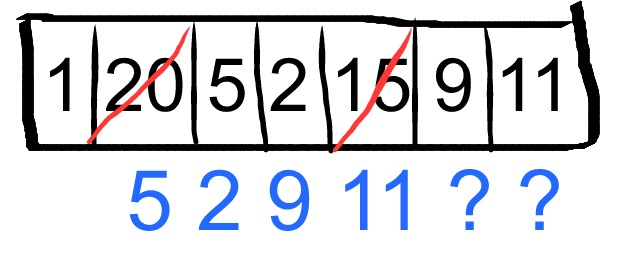The holes at the end will be erase by a vector member function - erase - because only the vector knows its internal details:

auto beginGarbage = remove_if(begin(v), end(v), [](int i) { return i>14; });
v.erase(beginGarbage, end(v));


Or, idiomatically:

v.erase(remove_if(begin(v), end(v), [](int i) { return i>14; }), end(v));


vector::erase provides an overload that physically erases a range of elements from the vector.

remove and remove_if are not the only algorithms behaving this way. unique is similar. It “removes” the adjacent equal values from a range. It does exactly what we need:

cin >> s;
cout << distance(unique(begin(s), end(s)), end(s)) << endl;


unique, just like remove_if, returns an iterator to the “new logical end” of the range. Then, we just need to measure the distance from the new end to the current. This is exactly the number duplicated adjacent characters: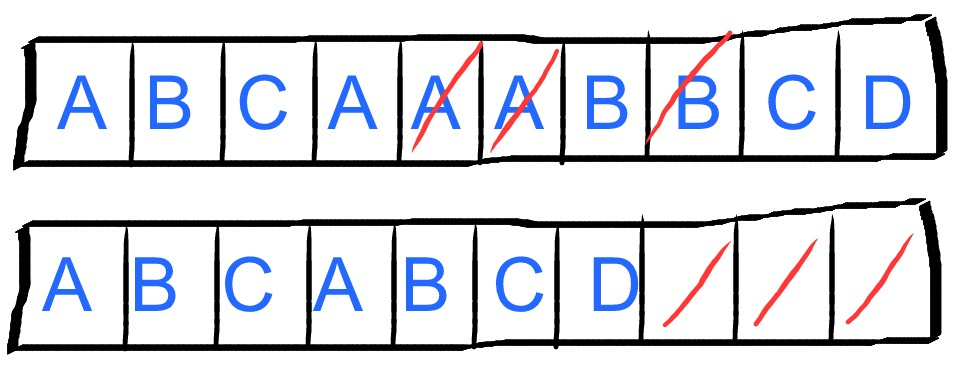What does it happen if we use unique and then erase on a sorted sequence?

Marco Arena, Last Edit: 9 May 2017